• 深度学习 PyTorch 一维卷积

千次阅读 2021-04-07 17:42:54
所谓的一维卷积，也就是卷积核是一维的。原理和2D Conv类似，只不过1D Conv的卷积核移动方向只有一个。 而且每组卷积核个数也等于输入人间的通道数。 实际操作中，以点云文件为例：包含nx3的数据，进行卷积前...
• 所谓的一维卷积，也就是卷积核是一维的。原理和2D Conv类似，只不过1D Conv的卷积核移动方向只有一个，从滑动窗口的直观角度来看，多通道一维卷积核在数据长度方向上滑动

• 而且每卷积核个数也等于输入数据的通道数。

• 设定多少的输出通道，就会有多少卷积核

• 实际操作中，以点云文件为例：包含nx3的数据，进行卷积前首先要交换样本个数与通道。

• ##代码备份##

import torch
import torch.nn as nn
from numpy import *

# Args:
#         in_channels (int): Number of channels in the input image  {输入通道数}
#         out_channels (int): Number of channels produced by the convolution  {输出通道}
#         kernel_size (int or tuple): Size of the convolving kernel
#         stride (int or tuple, optional): Stride of the convolution. Default: 1
#             the input. Default: 0
#         padding_mode (string, optional): 'zeros', 'reflect',
#             'replicate' or 'circular'. Default: 'zeros'
#         dilation (int or tuple, optional): Spacing between kernel
#             elements. Default: 1
#         groups (int, optional): Number of blocked connections from input
#             channels to output channels. Default: 1
#         bias (bool, optional): If True, adds a learnable bias to the
#             output. Default: True
#

conv1 = nn.Conv1d(3, 2, 2, 1)  # in, out, k_size, stride

a = torch.ones(1, 5, 3)  # b_size, n, 3  # 点云格式
a = a.permute(0, 2, 1)
# a = torch.ones(3)
print('a:\n', a)

b = conv1(a)
print('b:\n', b)
print(b.shape)  # b_size, ch, length

展开全文卷积神经网络 深度学习
• 刚刚接触到深度学习，前2个月的时间里，我用一维卷积神经网络实现了对于一维数据集的分类和回归。由于在做这次课题之前，我对深度学习基本上没有过接触，所以期间走了很多弯路。 在刚刚收到题目的要求时，我选择...

刚刚接触到深度学习，前2个月的时间里，我用一维的卷积神经网络实现了对于一维数据集的分类和回归。由于在做这次课题之前，我对深度学习基本上没有过接触，所以期间走了很多弯路。

在刚刚收到题目的要求时，我选择使用TensorFlow来直接编写函数，结果由于没有什么基础，写了一个周我就放弃了，改用keras来完成我的任务。

用keras来搭建神经网络其实很简单。我把自己的网络模型和数据集分享给大家，一起交流一起进步。

我们要完成的任务是对一些给定的湿度特征数据进行分类，多分类就是最简单最入门的深度学习案例。

我们要完成分类任务的数据集，247*900大小的一个矩阵，其中包含900条湿度信息，每条湿度信息由246位比特构成，最后一位表示湿度的类别，数值1-9，也就是每种湿度有100条。大家可以点击下边的链接自取。

数据集-用做分类.csv

或者百度网盘：

链接：https://pan.baidu.com/s/1tZlpRVBjRoGe2jHTKwRm6A
提取码：bm94

首先是数据的导入：

#载入数据

X = np.expand_dims(df.values[:, 0:246].astype(float), axis=2)
Y = df.values[:, 246]

X是900条数据的特征信息，Y表示真实类别。特别注意：这里的X在读取的时候矩阵增加了一维。使用一维卷积神经网络Conv1D的时候必须要事先这样对数据集进行处理，而只使用深度网络Dense时不需要额外的增加一维。
具体细节大家可以看我之前写过的一篇博客，比较细的区分了这两种情况：

Conv1D层与Dense层的连接
没有接触过的读者可以查一下只使用深度层和使用卷积层的区别。CNN眼里只有局部小特征，而DNN只有全局大特征。

最普通的深层神经网络包含多层神经元，从输入信号中提取信息。每个神经元接受来自前一层神经元的输入，并通过权重和非线性将它们组合起来。与普通神经网络中每个神经元与前一层的所有神经元连接不同，CNN中的每个神经元只与前一层的少数神经元局部连接。而且，CNN同一层的所有神经元都有相同的权重。CNN层中神经元的值通常被称为特征映射（features maps），因为它们可以被看作是对应于输入的不同部分的特征。卷积神经网络可以很好地捕获出原数据中的局部简单特征，随着层数的增加，产生的特征映射会捕获输入信号越来越多的全局和更复杂的属性。它可以在一条数据中较短的片段中获得感兴趣的特征，并且该特征在该数据片段中的位置不具有高度相关性

紧接着是对湿度类别的编码：

# 湿度分类编码为数字
encoder = LabelEncoder()
Y_encoded = encoder.fit_transform(Y)
Y_onehot = np_utils.to_categorical(Y_encoded)

Y_onehot编码是为了让程序自己来区分每一条特征信息而添加的编码。一个简单的例子解释一下：

这一步转化工作我们可以利用keras中的np_utils.to_categorical函数来进行。
“one-hot编码。one-hot编码的定义是用N位状态寄存器来对N个状态进行编码。比如[0,…,0]，[1,…,1]，[2,…,2]有3个分类值,因此N为3,对应的one-hot编码可以表示为100,010,001。”

我们将原始的数据集划分为训练集和测试集：

# 划分训练集，测试集
X_train, X_test, Y_train, Y_test = train_test_split(X, Y_onehot, test_size=0.3, random_state=0)

我这里把原始数据集以7：3的比例划分为训练集和测试集。训练集用来逼近真实类别，测试集来测试效果。
接着的是网络模型的搭建：

# 定义神经网络
def baseline_model():
model = Sequential()
plot_model(model, to_file='./model_classifier.png', show_shapes=True) # 保存网络结构为图片，这一步可以直接去掉
print(model.summary()) # 显示网络结构
return model

这一步往往是最核心的一步，网络模型通过卷积层来提取特征，在分类任务中，网络的最后一层为每个类。分类算法得到的是一个决策面，通过对比湿度信息属于每一类湿度的概率，进而对号入座。

经过多次调参尝试，最后我使用7层Conv1D来提取特征值，每两层Conv1D后添加一层MaxPooling1D来保留主要特征，减少计算量。每层卷积层使用双曲正切函数tanh（hyperbolic tangent function）来提高神经网络对模型的表达能力。tanh经常被运用到多分类任务中用做激活函数。

神经网络本就具有不可解释性，一般卷积核和全连接的结点数按照2的指数次幂来取。

Flatten()层作为中间层来链接卷积神经网络和全连接层。

神经网络被训练来最小化一个损失函数（Softmax loss），该函数捕捉到网络的特征输出与给定的期望输出之间的差异。9个输出结点对应九个类别的湿度，Softmax将每个特征数据匹配到概率最大的特征类别。

交叉熵损失函数（categorical crossentropy）作为模型训练的损失函数，它刻画的是当前学习到的概率分布与实际概率分布的距离，也就是损失函数越小，两个概率分布越相似，此时损失函数接近于0。

我将网络模型绘制成了AlexNet风格的示意图：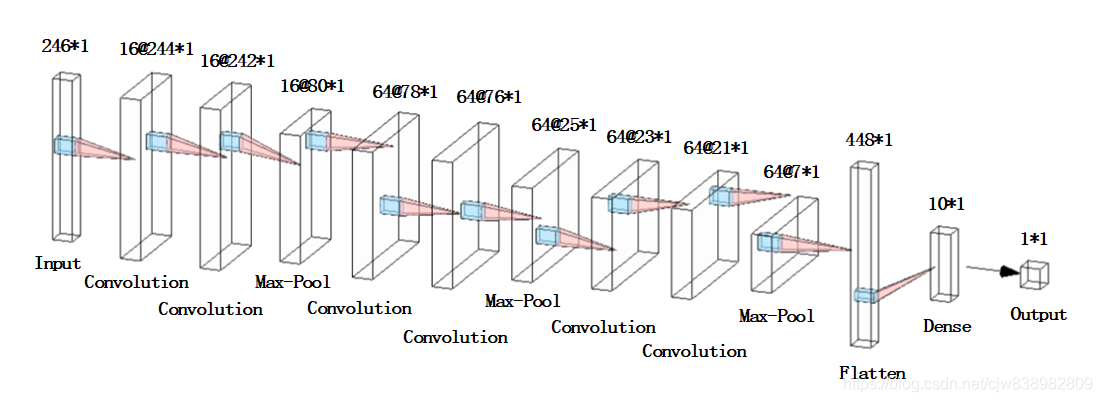这里也给大家安利下这个很好用的绘制神经网络的工具：

http://alexlenail.me/NN-SVG/AlexNet.html

搭建好网络之后就可以开始训练了。

# 训练分类器
estimator = KerasClassifier(build_fn=baseline_model, epochs=40, batch_size=1, verbose=1)
estimator.fit(X_train, Y_train)

batch_size设为1，然后一共训练40期。

这些数据大家都可以根据自己的实际情况做出调整和优化。

到这一步已经是搭建和训练的部分全部结束了。

紧接着是测试集来验证训练的准确性。

为了每次测试前不重复训练，我们将训练的模型保存下来：

# 将其模型转换为json
model_json = estimator.model.to_json()
with open(r"C:\Users\Desktop\model.json",'w')as json_file:
json_file.write(model_json)# 权重不在json中,只保存网络结构
estimator.model.save_weights('model.h5')

下面是读取模型的部分，这一部分完全可以写到另一个函数里：

# 加载模型用做预测
json_file = open(r"C:\Users\Desktop\model.json", "r")
json_file.close()
# 分类准确率
print("The accuracy of the classification model:")
print('%s: %.2f%%' % (loaded_model.metrics_names, scores * 100))
# 输出预测类别
print("predicted label:\n " + str(predicted_label))

当然我们也希望可以直观的显示出模型的分类精度，和训练的过程。

混淆矩阵经常用来表示分类的效果。

#显示混淆矩阵
plot_confuse(estimator.model, X_test, Y_test)

# 混淆矩阵定义
def plot_confusion_matrix(cm, classes,title='Confusion matrix',cmap=plt.cm.jet):
cm = cm.astype('float') / cm.sum(axis=1)[:, np.newaxis]
plt.imshow(cm, interpolation='nearest', cmap=cmap)
plt.rcParams['font.sans-serif'] = ['SimHei']  # 用来正常显示中文标签
plt.rcParams['axes.unicode_minus'] = False  # 用来正常显示负号
plt.colorbar()
tick_marks = np.arange(len(classes))
plt.xticks(tick_marks,('0%','3%','5%','8%','10%','12%','15%','18%','20%','25%'))
plt.yticks(tick_marks,('0%','3%','5%','8%','10%','12%','15%','18%','20%','25%'))
thresh = cm.max() / 2.
for i, j in itertools.product(range(cm.shape), range(cm.shape)):
plt.text(j, i, '{:.2f}'.format(cm[i, j]), horizontalalignment="center",color="white" if cm[i, j] > thresh else "black")
plt.tight_layout()
plt.ylabel('真实类别')
plt.xlabel('预测类别')
plt.savefig('test_xx.png', dpi=200, bbox_inches='tight', transparent=False)
plt.show()

# 显示混淆矩阵
def plot_confuse(model, x_val, y_val):
predictions = model.predict_classes(x_val)
truelabel = y_val.argmax(axis=-1)   # 将one-hot转化为label
conf_mat = confusion_matrix(y_true=truelabel, y_pred=predictions)
plt.figure()
plot_confusion_matrix(conf_mat, range(np.max(truelabel)+1))

如果好奇的话你也可以看看每层卷积层到底学到了什么：

# 可视化卷积层
visual(estimator.model, X_train, 1)

但是我觉得看一维卷积层的特征没啥意义，毕竟都是一些点，看起来有点非人类。

# 卷积网络可视化
def visual(model, data, num_layer=1):
# data:图像array数据
# layer:第n层的输出
layer = keras.backend.function([model.layers.input], [model.layers[num_layer].output])
f1 = layer([data])
print(f1.shape)
num = f1.shape[-1]
print(num)
plt.figure(figsize=(8, 8))
for i in range(num):
plt.subplot(np.ceil(np.sqrt(num)), np.ceil(np.sqrt(num)), i+1)
plt.imshow(f1[:, :, i] * 255, cmap='gray')
plt.axis('off')
plt.show()

最后运行的所有结果如下：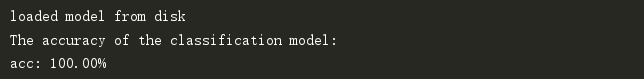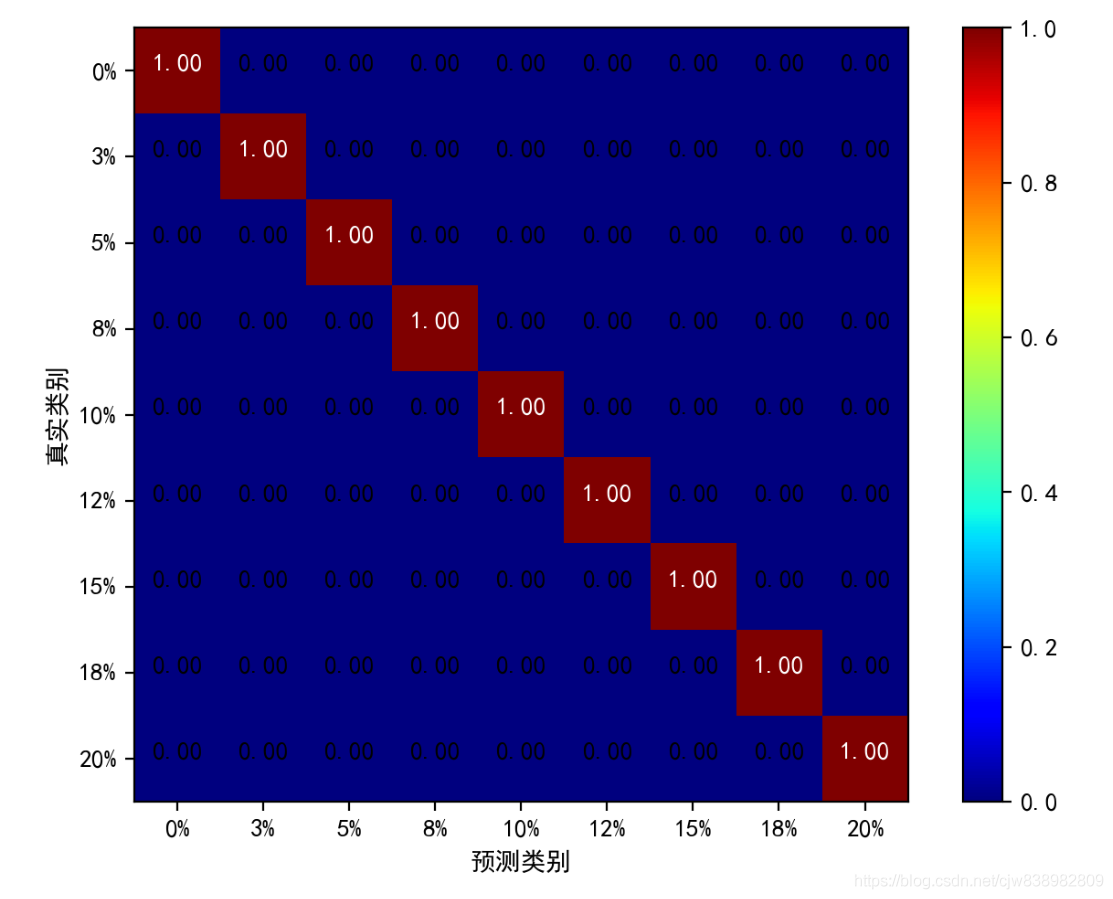这个结果这么好纯粹是因为数据比较好，分类的准确率很大程度都是依赖你数据集本身的区分度的。

下面是数据集的特征图，从图上就可以看出数据的层次性很直观。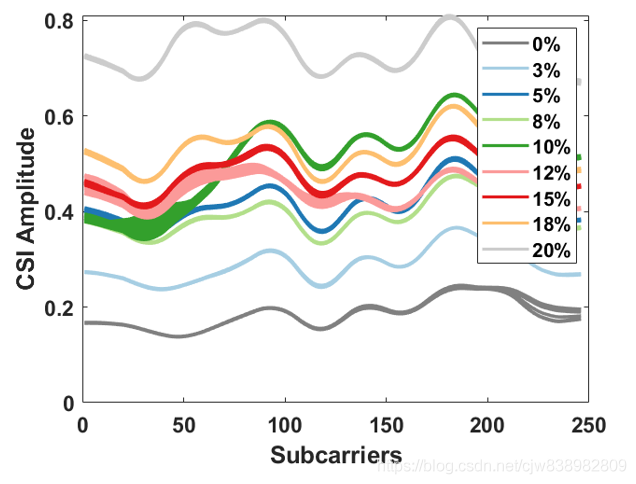当然我给大家上传的这部分数据是我这边结果最好的一批，其他数据集准确度达不到这么高。

下面贴出整个分类过程的完整代码：

# -*- coding: utf8 -*-
import numpy as np
import pandas as pd
import keras
from keras.models import Sequential
from keras.wrappers.scikit_learn import KerasClassifier
from keras.utils import np_utils,plot_model
from sklearn.model_selection import cross_val_score,train_test_split,KFold
from sklearn.preprocessing import LabelEncoder
from keras.layers import Dense,Dropout,Flatten,Conv1D,MaxPooling1D
from keras.models import model_from_json
import matplotlib.pyplot as plt
from sklearn.metrics import confusion_matrix
import itertools

# 载入数据
X = np.expand_dims(df.values[:, 0:246].astype(float), axis=2)
Y = df.values[:, 246]

# 湿度分类编码为数字
encoder = LabelEncoder()
Y_encoded = encoder.fit_transform(Y)
Y_onehot = np_utils.to_categorical(Y_encoded)

# 划分训练集，测试集
X_train, X_test, Y_train, Y_test = train_test_split(X, Y_onehot, test_size=0.3, random_state=0)

# 定义神经网络
def baseline_model():
model = Sequential()
plot_model(model, to_file='./model_classifier.png', show_shapes=True)
print(model.summary())
return model

# 训练分类器
estimator = KerasClassifier(build_fn=baseline_model, epochs=40, batch_size=1, verbose=1)
estimator.fit(X_train, Y_train)

# 卷积网络可视化
# def visual(model, data, num_layer=1):
#     # data:图像array数据
#     # layer:第n层的输出
#     layer = keras.backend.function([model.layers.input], [model.layers[num_layer].output])
#     f1 = layer([data])
#     print(f1.shape)
#     num = f1.shape[-1]
#     print(num)
#     plt.figure(figsize=(8, 8))
#     for i in range(num):
#         plt.subplot(np.ceil(np.sqrt(num)), np.ceil(np.sqrt(num)), i+1)
#         plt.imshow(f1[:, :, i] * 255, cmap='gray')
#         plt.axis('off')
#     plt.show()

# 混淆矩阵定义
def plot_confusion_matrix(cm, classes,title='Confusion matrix',cmap=plt.cm.jet):
cm = cm.astype('float') / cm.sum(axis=1)[:, np.newaxis]
plt.imshow(cm, interpolation='nearest', cmap=cmap)
plt.rcParams['font.sans-serif'] = ['SimHei']  # 用来正常显示中文标签
plt.rcParams['axes.unicode_minus'] = False  # 用来正常显示负号
plt.colorbar()
tick_marks = np.arange(len(classes))
plt.xticks(tick_marks,('0%','3%','5%','8%','10%','12%','15%','18%','20%','25%'))
plt.yticks(tick_marks,('0%','3%','5%','8%','10%','12%','15%','18%','20%','25%'))
thresh = cm.max() / 2.
for i, j in itertools.product(range(cm.shape), range(cm.shape)):
plt.text(j, i, '{:.2f}'.format(cm[i, j]), horizontalalignment="center",color="white" if cm[i, j] > thresh else "black")
plt.tight_layout()
plt.ylabel('真实类别')
plt.xlabel('预测类别')
plt.savefig('test_xx.png', dpi=200, bbox_inches='tight', transparent=False)
plt.show()

# seed = 42
# np.random.seed(seed)
# kfold = KFold(n_splits=10, shuffle=True, random_state=seed)
# result = cross_val_score(estimator, X, Y_onehot, cv=kfold)
# print("Accuracy of cross validation, mean %.2f, std %.2f\n" % (result.mean(), result.std()))

# 显示混淆矩阵
def plot_confuse(model, x_val, y_val):
predictions = model.predict_classes(x_val)
truelabel = y_val.argmax(axis=-1)   # 将one-hot转化为label
conf_mat = confusion_matrix(y_true=truelabel, y_pred=predictions)
plt.figure()
plot_confusion_matrix(conf_mat, range(np.max(truelabel)+1))

# 将其模型转换为json
model_json = estimator.model.to_json()
with open(r"C:\Users\316CJW\Desktop\毕设代码\model.json",'w')as json_file:
json_file.write(model_json)# 权重不在json中,只保存网络结构
estimator.model.save_weights('model.h5')

# 加载模型用做预测
json_file = open(r"C:\Users\Desktop\model.json", "r")
json_file.close()
# 分类准确率
print("The accuracy of the classification model:")
print('%s: %.2f%%' % (loaded_model.metrics_names, scores * 100))
# 输出预测类别
print("predicted label:\n " + str(predicted_label))
#显示混淆矩阵
plot_confuse(estimator.model, X_test, Y_test)

# 可视化卷积层
# visual(estimator.model, X_train, 1)

但是，湿度值到底是连续的数值，分类任务验证了我们数据集特征的可分辨性。

下一篇博客中，我将对数据集稍作修改，将湿度类别改为真实湿度值。

利用卷积神经网络来提取特征，实现线性回归，二者同出一脉。

【keras】一维卷积神经网络做回归
https://blog.csdn.net/cjw838982809/article/details/106871107

展开全文• 本帖最后由 yexu55066 于 2016-8-16 02:17 编辑我用的DeepLearnToolbox的代码改了改成一维卷积神经网络，来识别一维的心电信号，训练样本40000均分成三类，训练后发现训练部分错误率就超高，到测试集就把所有信号都...

本帖最后由 yexu55066 于 2016-8-16 02:17 编辑

我用的DeepLearnToolbox的代码改了改成一维卷积神经网络，来识别一维的心电信号，训练样本40000均分成三类，训练后发现训练部分错误率就超高，到测试集就把所有信号都分作了其中一类，各种参数卷积核大小学习率什么的怎么修改都没变化。

求问出现这种情况可能存在的原因还有解决方案？或者有没有可以直接用的一维卷积神经网络能发给我试试(550669220@qq.com)？谢谢大家啦

这是做出来的训练部分错误率图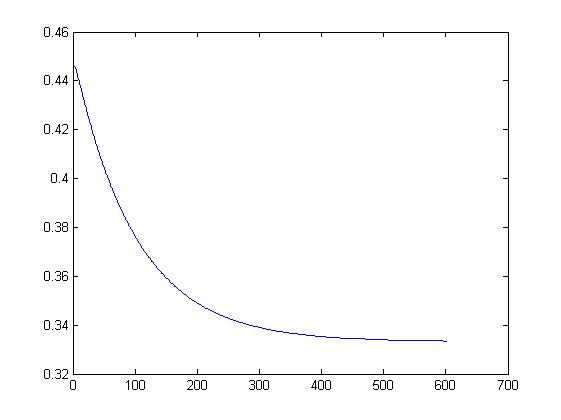trainerror.jpg (14.88 KB, 下载次数: 19)

2016-8-16 01:45 上传

然后下面是修改部分的代码。cnnsetup:

if strcmp(net.layers{l}.type, 'c')

mapsize(1) = mapsize(1) - net.layers{l}.kernelsize + 1;

fan_out = net.layers{l}.outputmaps * net.layers{l}.kernelsize;  %要学习的权值的数量

for j = 1 : net.layers{l}.outputmaps  %  output map

fan_in = inputmaps * net.layers{l}.kernelsize;            %多少参数连接到前一层

for i = 1 : inputmaps  %  input map

net.layers{l}.k{i}{j} =(rand(net.layers{l}.kernelsize,1) - 0.5) * 2 * sqrt(6 / (fan_in + fan_out));

cnnff

for l = 2 : n

if strcmp(net.layers{l}.type, 'c')

for j = 1 : net.layers{l}.outputmaps   %  for each output map

z = zeros(size(net.layers{l - 1}.a{1}) - [net.layers{l}.kernelsize-1 0 0]);

for j = 1 : inputmaps

z = convn(net.layers{l - 1}.a{j}, ones(net.layers{l}.scale,1) / (net.layers{l}.scale), 'valid');   %  !! replace with variable

net.layers{l}.a{j} = z(1 : net.layers{l}.scale : end, 1, :);

cnnbp

net.layers{l}.d{j} = net.layers{l}.a{j} .* (1 - net.layers{l}.a{j}) .* (expand(net.layers{l + 1}.d{j}, [net.layers{l + 1}.scale 1 1]) / net.layers{l + 1}.scale);

展开全文• 卷积神经网络（CNN）是深度学习中常用的网络架构，在智能语音...应用在图像问题上的CNN通常是二维卷积（因为图像有RGB三个通道），而语音问题上的CNN输入是一个矩阵平面，可以用一维卷积。本文就讲讲一维卷积是怎么处理

卷积神经网络（CNN）是深度学习中常用的网络架构，在智能语音中也不例外，比如语音识别。语音中是按帧来处理的，每一帧处理完就得到了相对应的特征向量，常用的特征向量有MFCC等，通常处理完一帧得到的是一个39维的MFCC特征向量。假设一段语音有N帧，处理完这段语音后得到的是一个39行N列（行表示特征维度，列表示帧数）的矩阵，这个矩阵是一个平面，是CNN的输入。应用在图像问题上的CNN通常是二维卷积（因为图像有RGB三个通道），而语音问题上的CNN输入是一个矩阵平面，可以用一维卷积。本文就讲讲一维卷积是怎么处理的。

所谓一维卷积是指卷积核只在一个方向上移动。具体到语音上，假设一段语音提取特征后是一个M行N列（M表示特征维度，N表示帧数）的矩阵平面，卷积核要在帧的方向上从小到大移动，下图给出了示意。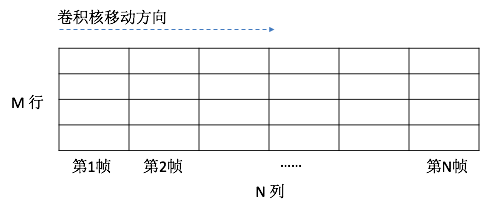卷积核也是一个矩阵（J行K列）。由于卷积核只在一个方向上移动，要把所有的特征值都覆盖到，必须卷积核的行数要等于特征值矩阵的行数，即J = M，所以描述卷积核时只需要知道kernel size（即多少列）和 kernel count（即多少个kernel）。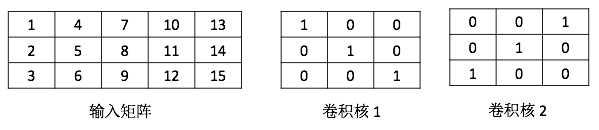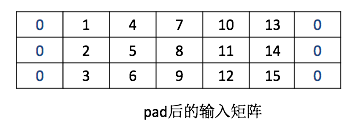做卷积时先从左边的padding处开始，具体如下图，相对应的位置相乘再相加后，再加上bias就可以了,计算出值为10(0 x 1 + 1 x 0 + 4 x 0 + 0 x 0 + 2 x 1 + 5 x 0 + 0 x 0 + 3 x 0 + 6 x 1 + 2 = 10)。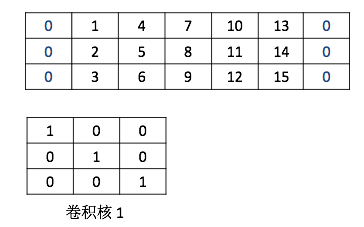把卷积核向帧增大方向（即向右）移动一格，如下图：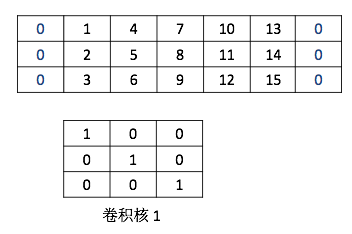同样方法计算出值为17(1 x 1 + 4 x 0 + 7 x 0 + 2 x 0 + 5 x 1 + 8 x 0 + 3 x 0 + 6 x 0 + 9 x 1 + 2 = 17)。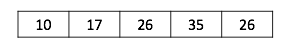第二个kernel的卷积计算与第一个kernel计算一样，得到的也是一个1行5列的矩阵。如下图：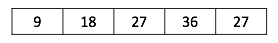最终一个3x5的输入矩阵与kernel count为2、kernel size为3的卷积后得到的是一个2行5列的矩阵，如下图。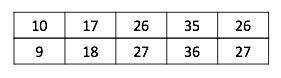以上就是一维卷积的处理过程，很简单的吧。

展开全文• 实战一维卷积(Conv1D)：kaggle叶子分类问题通过例子理解：(1)一维卷积是如何工作的；(2)输入数据是如何构建的；在csv文件中，数据集或者特征集的保存方式nxm，其中n为样本个数，m为特征个数。这里有3类特征，64个...一维卷积filter
• 一维卷积和二维卷积的维度是指，输入数据的维度，比如一维的只有长度（如文本，第一个单词第二个单词），二维的有长宽（如图像，横纵分布的像素矩阵）。 一维卷积的卷积核在一维方向上移动 （如文本，第一个单词第...深度学习 pytorch 神经网络
• 首先要强调一点，一维卷积的含义是卷积核移动的方向是一维的，数据可以是二维的，很多初学者容易混淆这个概念一维卷积 tensorflow 深度学习 RNN
• 在TensorFlow学习笔记(8):CNN实现中我们以图像处理为场景对卷积神经网络进行了介绍，包括了卷积操作和pooling操作的实现，其中卷积和pooling都采用了二的操作，输入数据是四的，shape = [batch, in_height, in_...
• 数据集与[PyTorch]手动实现二维卷积神经网络完成车辆分类任务相同。本篇对于数据的处理以及模型的训练测试与上文相同，主要放上利用torch.nn实现二维卷积模型的代码。 （）模型的构建 # 使用torch.nn实现二维卷积 ...卷积
• 基于多通道的一维卷积神经网络故障诊断

千次阅读 热门讨论 2021-04-07 15:38:09
、数据准备 本次采用的PHM 2009 Challenge Data的全套齿轮箱数据集进行实验分析，包括齿轮、轴承以及轴的故障。其实验平台如下图所示： 本次选用50HZ的转速下低负载通道1的振动信号数据进行构建数据集，每类样本...
• 文章目录（）实验任务（二）...手写二维卷积的实现，并在车辆分类数据集上完成分类任务 数据集包含三个文件夹，里面分别是car、bus和truck的车辆图片，需要对数据集进行处理并且划分数据集。 （二）数据处理 数据形式卷积 python
• 然后用16个5*5的卷积核进行卷积 self.conv2 = nn.Conv2d(6, 16, 5) # 添加全连接层，进行分类，也就是将卷积后的数据展开，为16个4*4的图像， # 下层的输入为上层的输出，所以16*4*4 self.liner_1 = nn.Linear(16*4*...卷积 深度学习 神经网络 python
• 要对输出进行分类，请包括个输出大小为 K 的全连接层、个 softmax 层和分类层，其中 K 是类的数量。 首先，在个层数组中，指定输入层、首个一元分词块、深度串联层、全连接层、softmax 层和分类层。 ...
• torch.nn.Conv1d及一维卷积详解

千次阅读 2021-02-02 11:04:45
最近在用一维卷积分类，所以看了一维卷积的API和各种博客。 主要的参考博客是（两个博客综合起来看很不错）： https://blog.csdn.net/qq_36323559/article/details/102937606 ... Conv1 ...class torch.nn.Conv1d(in_...
• 计算方法和二维卷积类似，从三维图像中划分出3×3×33\times3\times33×3×3的方块（称为卷积立方体），对这27个像素点进行卷积，即逐个元素与过滤器相乘求和，得到输出二维矩阵中的个值。 三维图像和过滤器可以...
• 为什么在 NLP 分类任务中选择 CNN 呢？ 1.CNN神经网络可以像处理图像一样处理文本并“理解”它们 2.主要好处是高效率 3.在许多方面，由于池化层和卷积核大小所造成的限制（虽然可以将卷积核设置得更大），会导致丢弃...卷积 神经网络 python 情感分析 nlp
• CNN一维卷积详解

千次阅读 2021-07-27 19:10:00
PyTorch中的nn.Conv1d与nn.Conv2d ... 32019.04.29 20:30:41字数 1,134阅读 62,663 ...一般来说，一维卷积nn.Conv1d用于文本数据，只对宽度进行卷积，对高度不卷积。通常，输入大小为word_embedding_dim * max_le
• 在用C语言实现图像处理中，经常要用到二维卷积的运算，这个在matlab中是非常容易实现的，只需要conv2()就OK啦，而且速度非常的快。但是在C语言中就需要四层的for循环来实现了。首先二维卷积的原理是：123 222A= 123B...
• 介绍生物医学信号在研究和数据科学领域始终发挥着重要作用。就卷积神经网络 (CNN) 而言，这种特殊算法在定义深度学习 (DL) 等最复杂和最高级算法的架构方面发挥着重要作用。谈到深度学习，...算法 神经网络 卷积 python 机器学习
• 点击上方“小白学视觉”，选择加"星标"或“置顶”重磅干货，第时间送达 本文转自 | 人工智能与算法学习概述许多文章都关注于二维卷积神经网络（2D CNN）的使用，特别是...神经网络 网络 python 计算机视觉 机器学习
• 1.什么是三维卷积 1.1 三维卷积简介 1.2 三维卷积的工作原理 2，三维卷积核多通道卷积的区别 2.1 多通道卷积 2.2 三维卷积和多通道卷积之间的区别 2.3 总结 3，三维卷积的应用 3.1 视频分类 3.2 点云分类 ...深度学习 计算机视觉 神经网络
• 据了解rest-net从图像分类大赛上表现优异，那么将rest-net进行降维用于一维数据分类会有怎样表现？ 先观察一下Rest-net的结构：由于每个截图有限，所以使用多个图片表现出?????????????????????????????????????????...深度学习 tensorflow 神经网络 机器学习
• 1.类的定义 import torch.nn as nn import torch class Basic...self.avgpool(x) x = torch.flatten(x, 1)#压平 变成一维矩阵 x = self.fc(x)#全连接 return x def resnet18(num_classes=1000, include_top=True): # ...python
• 2.卷积过程可以看做翻折后的h［］序列在x［］序列上移动对应项相乘求和的过程，而求和过程的分类可以分为三类情况：h［］进入x［］移动的过程，h［］完全进入x［］移动的过程，h［］出x［］移动的过程。 3.这三种...
• 、pandas是什么？ 二、使用步骤 1.引入库 2.读入数据 总结 前言 提示：这里可以添加本文要记录的大概内容： 例如：随着人工智能的不断发展，机器学习这门技术也越来越重要，很多人都开启了学习机器...python 人工智能 卷积神经网络 tensorflow 深度学习
• 我有个由不同长度的时间序列组成的数据集。例如，考虑一下这个ts1 = np.random.rand(230, 4)ts2 = np.random.rand(12309, 4)我有200个数组列表形式的序列^{pr2}\$如果良好，这些时间序列的标签为1，否则为0。因此我...
• 文章目录内容介绍使用智能手机数据集进行活动识别开发一维卷积神经网络拟合和评估模型总结结果调整一维卷积神经网络过滤器数量内核大小多头卷积神经网络一些拓展的想法 内容介绍 人类活动识别是将专用线束或智能手机...
• 点击蓝字关注我们AI TIME欢迎每位AI爱好者的加入！普通的神经网络不适用于比较大的网格（因为参数会过多），而传统的卷积神经网络又没法应用在拥有不规则结构的三网格上。因此，我们把传...卷积 神经网络 网络 算法 编程语言
• 众多师弟师妹在使用一维卷积神经网络对凯斯西储，哥伦比亚等大学开源的轴承故障数据集进行故障诊断时，不知道该怎么把Matlab文件中的数据直接导进伸进网络进行训练。 我之前也不会，我一直用matlab打开数据然后黏贴...神经网络 matlab python
• 这篇文章的任务是预测给定社交网络本身每个成员倾向于加入哪社区（二分类问题）。 karate_club_graph 是 networkx 模块集成的个数据集，这是社会网络分析领域中的经典数据集，20世纪70年代，社会学家 Zachary ...深度学习 神经网络 python 图卷积 分类算法...